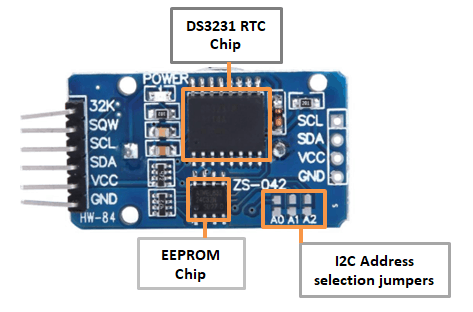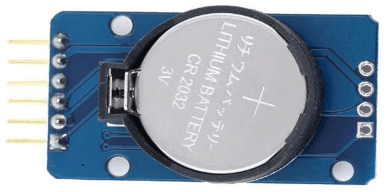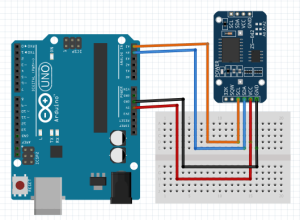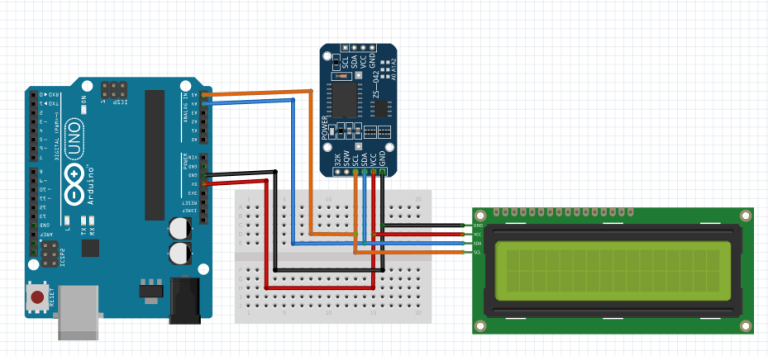# DS3231 RTC interfacing with Arduino.Table of Contents

The DS3231 RTC module is among the best for microcontroller projects involving data-logging, clock-building, time stamping, timers and alarms. In this tutorial we shall show how this module works and how to interface it with Arduino for keeping time.
In a previous tutorial I talked about the DS1307 RTC which you can refer to :
• How to use DS1307 Real Time Clock with Arduino
• ## Structure of the DS3231 RTC.The major coponent of this RTC module is the DS3231 chip which is a low-cost and extremely accurate RTC chip. It is responsible for all timekeeping functions and can easily be interfaced with any microcontroller via the I2C protocol.
The DS3231 rtc is driven by a 32kHz Temperature Compensated Crystal Oscillator (TCXO) which is packaged inside the chip.This Crystal Oscillator is highly immune to the external temperature changes that often affect oscillation frequency and this is the reason why the module is more accurate at time keeping than other RTC modules.
Right next to the integrated crystal is a temperature sensor which compensates the frequency changes by adding or removing clock ticks so that the timekeeping stays on track.
The on board 32 bytes 24C32 EEPROM chip can be used to save settings or really anything.The 24C32 EEPROM uses I2C interface for communication and shares the same I2C bus as DS3231. The I2C address of the EEPROM can be changed easily with the three A0, A1 and A2 solder jumpers at the back. Each one of these is used to hardcode in the address. If a jumper is shorted with solder, that sets the address.
There is a battery backup at bottom side of the board that holds a 20mm 3V lithium coincell (any CR2032 battery can fit well) for maintaining accurate timekeeping when main power to the device is interrupted. So you don’t need to worry about power outages, your MCU can still keep track of time.

## Setting time of the DS3231 RTC

Before using the DS3231 rtc module, we need to first update it’s time and date settings. This is done by connecting the RTC module to an Arduino board and using already made libraries, we can be able to see the set time and date on the serial monitor. The RTClib.h library is easy to use because it will automatically set the current date and time using the time on your computer.The DS3231 RTC will be connected to the Arduino Uno board as follows:
SCL to Pin A5
SDA to Pin A4
GND to Arduino ground
VCC to Arduino 5V.

### Code

This code uses the RTClib.h library. When uploaded to the Arduino board with a DS3231 RTC module connected as shown above, the current time and date are automatically displayed on the serial monitor.The time and date displayed will be got automatically from the device you are using with the Arduino IDE for example a computer.
```#include "RTClib.h"
RTC_DS3231 rtc;
char daysOfTheWeek = {"Sunday", "Monday", "Tuesday", "Wednesday", "Thursday", "Friday", "Saturday"};

void setup () {
#ifndef ESP8266
while (!Serial); // for Leonardo/Micro/Zero
#endif
Serial.begin(9600);
delay(3000); // wait for console opening

if (! rtc.begin()) {
Serial.println("Couldn't find RTC");
while (1);
}

if (rtc.lostPower()) {
Serial.println("RTC lost power, lets set the time!");
// following line sets the RTC to the date & time this sketch was compiled
rtc.adjust(DateTime(F(__DATE__), F(__TIME__)));
// This line sets the RTC with an explicit date & time, for example to set
// January 21, 2014 at 3am you would call:
// rtc.adjust(DateTime(2014, 1, 21, 3, 0, 0));
}
}

void loop () {
DateTime now = rtc.now();

Serial.print(now.year(), DEC);
Serial.print('/');
Serial.print(now.month(), DEC);
Serial.print('/');
Serial.print(now.day(), DEC);
Serial.print(" (");
Serial.print(daysOfTheWeek[now.dayOfTheWeek()]);
Serial.print(") ");
Serial.print(now.hour(), DEC);
Serial.print(':');
Serial.print(now.minute(), DEC);
Serial.print(':');
Serial.print(now.second(), DEC);
Serial.println();

Serial.print(" since midnight 1/1/1970 = ");
Serial.print(now.unixtime());
Serial.print("s = ");
Serial.print(now.unixtime() / 86400L);
Serial.println("d");

// calculate a date which is 7 days and 30 seconds into the future
DateTime future (now + TimeSpan(7,12,30,6));

Serial.print(" now + 7d + 30s: ");
Serial.print(future.year(), DEC);
Serial.print('/');
Serial.print(future.month(), DEC);
Serial.print('/');
Serial.print(future.day(), DEC);
Serial.print(' ');
Serial.print(future.hour(), DEC);
Serial.print(':');
Serial.print(future.minute(), DEC);
Serial.print(':');
Serial.print(future.second(), DEC);
Serial.println();

Serial.print("Temperature: ");
Serial.print(rtc.getTemperature());
Serial.println(" C");

Serial.println();
delay(3000);
}

```
The time and date can also be set manually using the code below. In this case we need to change the setup part of the code where the time and date are set from this line of code below.
``` parse_cmd("T300608330032016",16);// this means 08:06:30 30.03.2016 //TssmmhhWDDMMYYYY(sec min hr day date month year) ```
After setting the time for the first time,the RTC stores the time and as such this line of code should be commented out for all other uses.
```#include "ds3231.h"
#include "rtc_ds3231.h"

#define BUFF_MAX 128

uint8_t time;
char recv[BUFF_MAX];
unsigned int recv_size = 0;
unsigned long prev, interval = 5000;

void setup()
{
Serial.begin(9600);
DS3231_init(DS3231_CONTROL_INTCN);
memset(recv, 0, BUFF_MAX);
Serial.println("GET time");

Serial.println("Setting time");
//parse_cmd("T303515617082019",16); //Displayed as 2019.08.17 15:35:34
//           TssmmhhWDDMMYYYY

}

void loop()
{
char in;
char buff[BUFF_MAX];
unsigned long now = millis();
struct ts t;

// show time once in a while
if ((now - prev > interval) && (Serial.available() <= 0)) {
DS3231_get(&t);

// there is a compile time option in the library to include unixtime support
#ifdef CONFIG_UNIXTIME
snprintf(buff, BUFF_MAX, "%d.%02d.%02d %02d:%02d:%02d %ld", t.year,
t.mon, t.mday, t.hour, t.min, t.sec, t.unixtime);
#else
snprintf(buff, BUFF_MAX, "%d.%02d.%02d %02d:%02d:%02d", t.year,
t.mon, t.mday, t.hour, t.min, t.sec);
#endif

Serial.println(buff);
prev = now;
}

if (Serial.available() > 0) {
in = Serial.read();

if ((in == 10 || in == 13) && (recv_size > 0)) {
parse_cmd(recv, recv_size);
recv_size = 0;
recv = 0;
} else if (in < 48 || in > 122) {;       // ignore ~[0-9A-Za-z]
} else if (recv_size > BUFF_MAX - 2) {   // drop lines that are too long
// drop
recv_size = 0;
recv = 0;
} else if (recv_size < BUFF_MAX - 2) {
recv[recv_size] = in;
recv[recv_size + 1] = 0;
recv_size += 1;
}

}
}

void parse_cmd(char *cmd, int cmdsize)
{
uint8_t i;
uint8_t reg_val;
char buff[BUFF_MAX];
struct ts t;

//snprintf(buff, BUFF_MAX, "cmd was '%s' %d\n", cmd, cmdsize);
//Serial.print(buff);

// TssmmhhWDDMMYYYY aka set time
if (cmd == 84 && cmdsize == 16) {
//T355720619112011
t.sec = inp2toi(cmd, 1);
t.min = inp2toi(cmd, 3);
t.hour = inp2toi(cmd, 5);
t.wday = cmd - 48;
t.mday = inp2toi(cmd, 8);
t.mon = inp2toi(cmd, 10);
t.year = inp2toi(cmd, 12) * 100 + inp2toi(cmd, 14);
DS3231_set(t);
Serial.println("OK");
} else if (cmd == 49 && cmdsize == 1) {  // "1" get alarm 1
DS3231_get_a1(&buff, 59);
Serial.println(buff);
} else if (cmd == 50 && cmdsize == 1) {  // "2" get alarm 1
DS3231_get_a2(&buff, 59);
Serial.println(buff);
} else if (cmd == 51 && cmdsize == 1) {  // "3" get aging register
Serial.print("aging reg is ");
Serial.println(DS3231_get_aging(), DEC);
} else if (cmd == 65 && cmdsize == 9) {  // "A" set alarm 1
DS3231_set_creg(DS3231_CONTROL_INTCN | DS3231_CONTROL_A1IE);
//ASSMMHHDD
for (i = 0; i < 4; i++) {
time[i] = (cmd[2 * i + 1] - 48) * 10 + cmd[2 * i + 2] - 48; // ss, mm, hh, dd
}
uint8_t flags = { 0, 0, 0, 0, 0 };
DS3231_set_a1(time, time, time, time, flags);
DS3231_get_a1(&buff, 59);
Serial.println(buff);
} else if (cmd == 66 && cmdsize == 7) {  // "B" Set Alarm 2
DS3231_set_creg(DS3231_CONTROL_INTCN | DS3231_CONTROL_A2IE);
//BMMHHDD
for (i = 0; i < 4; i++) {
time[i] = (cmd[2 * i + 1] - 48) * 10 + cmd[2 * i + 2] - 48; // mm, hh, dd
}
uint8_t flags = { 0, 0, 0, 0 };
DS3231_set_a2(time, time, time, flags);
DS3231_get_a2(&buff, 59);
Serial.println(buff);
} else if (cmd == 67 && cmdsize == 1) {  // "C" - get temperature register
Serial.print("temperature reg is ");
Serial.println(DS3231_get_treg(), DEC);
} else if (cmd == 68 && cmdsize == 1) {  // "D" - reset status register alarm flags
reg_val = DS3231_get_sreg();
reg_val &= B11111100;
DS3231_set_sreg(reg_val);
} else if (cmd == 70 && cmdsize == 1) {  // "F" - custom fct
reg_val = DS3231_get_addr(0x5);
Serial.print("orig ");
Serial.print(reg_val,DEC);
Serial.print("month is ");
Serial.println(bcdtodec(reg_val & 0x1F),DEC);
} else if (cmd == 71 && cmdsize == 1) {  // "G" - set aging status register
DS3231_set_aging(0);
} else if (cmd == 83 && cmdsize == 1) {  // "S" - get status register
Serial.print("status reg is ");
Serial.println(DS3231_get_sreg(), DEC);
} else {
Serial.print("unknown command prefix ");
Serial.println(cmd);
Serial.println(cmd, DEC);
}
}

```

## DS3231 RTC with Arduino and LCD

We can now display the time, date and temperature on an LCD screen and the connection is as shown below. In this case we shall use an I2C LCD display.### Code

```#include <Wire.h>
#include "ds3231.h"
#include <LiquidCrystal_I2C.h>

//I2C pins declaration
LiquidCrystal_I2C lcd(0x27, 2, 1, 0, 4, 5, 6, 7, 3, POSITIVE);

#define BUFF_MAX 128

uint8_t time;
char recv[BUFF_MAX];
unsigned int recv_size = 0;
unsigned long prev, interval = 1000;

void setup()
{
Serial.begin(9600);
Wire.begin();
DS3231_init(DS3231_CONTROL_INTCN);
memset(recv, 0, BUFF_MAX);
Serial.println("GET time");
lcd.begin(20, 4);
lcd.backlight();//To Power ON the back light
lcd.clear();

//Serial.println("Setting time");
//parse_cmd("T300608330032016",16);
//           TssmmhhWDDMMYYYY
}

void loop()
{
char in;
char tempF;
float temperature;
char buff[BUFF_MAX];
unsigned long now = millis();
struct ts t;

// show time once in a while
if ((now - prev > interval) && (Serial.available() <= 0)) {
DS3231_get(&t); //Get time
parse_cmd("C",1);
temperature = DS3231_get_treg(); //Get temperature
dtostrf(temperature, 5, 1, tempF);

lcd.clear();
lcd.setCursor(1,0);

lcd.print(t.mday);

printMonth(t.mon);

lcd.print(t.year);

lcd.setCursor(0,1); //Go to second line of the LCD Screen
lcd.print(t.hour);
lcd.print(":");
if(t.min<10)
{
lcd.print("0");
}
lcd.print(t.min);
lcd.print(":");
if(t.sec<10)
{
lcd.print("0");
}
lcd.print(t.sec);

lcd.print(' ');
lcd.print(tempF);
lcd.print((char)223);
lcd.print("C ");
prev = now;
}

if (Serial.available() > 0) {
in = Serial.read();

if ((in == 10 || in == 13) && (recv_size > 0)) {
parse_cmd(recv, recv_size);
recv_size = 0;
recv = 0;
} else if (in < 48 || in > 122) {;       // ignore ~[0-9A-Za-z]
} else if (recv_size > BUFF_MAX - 2) {   // drop lines that are too long
// drop
recv_size = 0;
recv = 0;
} else if (recv_size < BUFF_MAX - 2) {
recv[recv_size] = in;
recv[recv_size + 1] = 0;
recv_size += 1;
}

}
}

void parse_cmd(char *cmd, int cmdsize)
{
uint8_t i;
uint8_t reg_val;
char buff[BUFF_MAX];
struct ts t;

//snprintf(buff, BUFF_MAX, "cmd was '%s' %d\n", cmd, cmdsize);
//Serial.print(buff);

// TssmmhhWDDMMYYYY aka set time
if (cmd == 84 && cmdsize == 16) {
//T355720619112011
t.sec = inp2toi(cmd, 1);
t.min = inp2toi(cmd, 3);
t.hour = inp2toi(cmd, 5);
t.wday = inp2toi(cmd, 7);
t.mday = inp2toi(cmd, 8);
t.mon = inp2toi(cmd, 10);
t.year = inp2toi(cmd, 12) * 100 + inp2toi(cmd, 14);
DS3231_set(t);
Serial.println("OK");
} else if (cmd == 49 && cmdsize == 1) {  // "1" get alarm 1
DS3231_get_a1(&buff, 59);
Serial.println(buff);
} else if (cmd == 50 && cmdsize == 1) {  // "2" get alarm 1
DS3231_get_a2(&buff, 59);
Serial.println(buff);
} else if (cmd == 51 && cmdsize == 1) {  // "3" get aging register
Serial.print("aging reg is ");
Serial.println(DS3231_get_aging(), DEC);
} else if (cmd == 65 && cmdsize == 9) {  // "A" set alarm 1
DS3231_set_creg(DS3231_CONTROL_INTCN | DS3231_CONTROL_A1IE);
//ASSMMHHDD
for (i = 0; i < 4; i++) {
time[i] = (cmd[2 * i + 1] - 48) * 10 + cmd[2 * i + 2] - 48; // ss, mm, hh, dd
}
byte flags = { 0, 0, 0, 0, 0 };
DS3231_set_a1(time, time, time, time, flags);
DS3231_get_a1(&buff, 59);
Serial.println(buff);
} else if (cmd == 66 && cmdsize == 7) {  // "B" Set Alarm 2
DS3231_set_creg(DS3231_CONTROL_INTCN | DS3231_CONTROL_A2IE);
//BMMHHDD
for (i = 0; i < 4; i++) {
time[i] = (cmd[2 * i + 1] - 48) * 10 + cmd[2 * i + 2] - 48; // mm, hh, dd
}
byte flags = { 0, 0, 0, 0 };
DS3231_set_a2(time, time, time, flags);
DS3231_get_a2(&buff, 59);
Serial.println(buff);
} else if (cmd == 67 && cmdsize == 1) {  // "C" - get temperature register
Serial.print("temperature reg is ");
Serial.println(DS3231_get_treg(), DEC);
} else if (cmd == 68 && cmdsize == 1) {  // "D" - reset status register alarm flags
reg_val = DS3231_get_sreg();
reg_val &= B11111100;
DS3231_set_sreg(reg_val);
} else if (cmd == 70 && cmdsize == 1) {  // "F" - custom fct
reg_val = DS3231_get_addr(0x5);
Serial.print("orig ");
Serial.print(reg_val,DEC);
Serial.print("month is ");
Serial.println(bcdtodec(reg_val & 0x1F),DEC);
} else if (cmd == 71 && cmdsize == 1) {  // "G" - set aging status register
DS3231_set_aging(0);
} else if (cmd == 83 && cmdsize == 1) {  // "S" - get status register
Serial.print("status reg is ");
Serial.println(DS3231_get_sreg(), DEC);
} else {
Serial.print("unknown command prefix ");
Serial.println(cmd);
Serial.println(cmd, DEC);
}
}

void printMonth(int month)
{
switch(month)
{
case 1: lcd.print(" January ");break;
case 2: lcd.print(" February ");break;
case 3: lcd.print(" March ");break;
case 4: lcd.print(" April ");break;
case 5: lcd.print(" May ");break;
case 6: lcd.print(" June ");break;
case 7: lcd.print(" July ");break;
case 8: lcd.print(" August ");break;
case 9: lcd.print(" September ");break;
case 10: lcd.print(" October ");break;
case 11: lcd.print(" November ");break;
case 12: lcd.print(" December ");break;
default: lcd.print(" Error ");break;
}
}

```
Back to top